# Inverting and Noninverting OpAmp Voltage Amplifier Circuits

• #### Question 1

 Don’t just sit there! Build something!!

Learning to mathematically analyze circuits requires much study and practice. Typically, students practice by working through lots of sample problems and checking their answers against those provided by the textbook or the instructor. While this is good, there is a much better way.

You will learn much more by actually building and analyzing real circuits, letting your test equipment provide the “answers” instead of a book or another person. For successful circuit-building exercises, follow these steps:

1. Carefully measure and record all component values prior to circuit construction.
2. Draw the schematic diagram for the circuit to be analyzed.
3. Carefully build this circuit on a breadboard or other convenient medium.
4. Check the accuracy of the circuit’s construction, following each wire to each connection point, and verifying these elements one-by-one on the diagram.
5. Mathematically analyze the circuit, solving for all voltage and current values.
6. Carefully measure all voltages and currents, to verify the accuracy of your analysis.
7. If there are any substantial errors (greater than a few percent), carefully check your circuit’s construction against the diagram, then carefully re-calculate the values and re-measure.

Avoid using the model 741 op-amp, unless you want to challenge your circuit design skills. There are more versatile op-amp models commonly available for the beginner. I recommend the LM324 for DC and low-frequency AC circuits, and the TL082 for AC projects involving audio or higher frequencies.

As usual, avoid very high and very low resistor values, to avoid measurement errors caused by meter “loading”. I recommend resistor values between 1 kΩ and 100 kΩ.

One way you can save time and reduce the possibility of error is to begin with a very simple circuit and incrementally add components to increase its complexity after each analysis, rather than building a whole new circuit for each practice problem. Another time-saving technique is to re-use the same components in a variety of different circuit configurations. This way, you won’t have to measure any component’s value more than once.

• #### Question 2

Write the transfer function (input/output equation) for an operational amplifier with an open-loop voltage gain of 100,000, and the inverting input connected directly to its output terminal. In other words, write an equation describing the output voltage of this op-amp (Vout) for any given input voltage at the non-inverting input (Vin(+)):Then, once you have an equation written, solve for the over-all voltage gain $$(A_v = \frac{V_{out}}{V_{in(+)}})$$ of this amplifier circuit, and calculate the output voltage for a non-inverting input voltage of 6 volts.

• #### Question 3

Write the transfer function (input/output equation) for an operational amplifier with an open-loop voltage gain of 100,000, and the inverting input connected to a voltage divider on its output terminal (so the inverting input receives exactly one-half the output voltage). In other words, write an equation describing the output voltage of this op-amp (Vout) for any given input voltage at the non-inverting input (Vin(+)):Then, once you have an equation written, solve for the output voltage if the non-inverting input voltage is -2.4 volts.

• #### Question 4

Generators used in battery-charging systems must be regulated so as to not overcharge the battery(ies) they are connected to. Here is a crude, relay-based voltage regulator for a DC generator: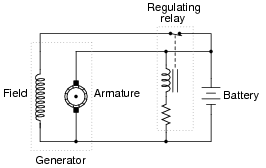Simple electromechanical relay circuits such as this one were very common in automotive electrical systems during the 1950’s, 1960’s, and 1970’s. The fundamental principle upon which their operation is based is called negative feedback: where a system takes action to oppose any change in a certain variable. In this case, the variable is generator output voltage. Explain how the relay works to prevent the generator from overcharging the battery with excessive voltage.

• #### Question 5

A mechanic has an idea for upgrading the electrical system in an automobile originally designed for 6 volt operation. He wants to upgrade the 6 volt headlights, starter motor, battery, etc, to 12 volts, but wishes to retain the original 6-volt generator and regulator. Shown here is the original 6-volt electrical system:The mechanic’s plan is to replace all the 6-volt loads with 12-volt loads, and use two 6-volt batteries connected in series, with the original (6-volt) regulator sensing voltage across only one of those batteries:Explain how this system is supposed to work. Do you think the mechanic’s plan is practical, or are there any problems with it?

• #### Question 6

Calculate the overall voltage gain of this amplifier circuit (AV), both as a ratio and as a figure in units of decibels (dB). Also, write a general equation for calculating the voltage gain of such an amplifier, given the resistor values of R1 and R2:• #### Question 7

Calculate all voltage drops and currents in this circuit, complete with arrows for current direction and polarity markings for voltage polarity. Then, calculate the overall voltage gain of this amplifier circuit (AV), both as a ratio and as a figure in units of decibels (dB):• #### Question 8

Calculate all voltage drops and currents in this circuit, complete with arrows for current direction and polarity markings for voltage polarity. Then, calculate the overall voltage gain of this amplifier circuit (AV), both as a ratio and as a figure in units of decibels (dB):• #### Question 9

Determine both the input and output voltage in this circuit:• #### Question 10

What would have to be altered in this circuit to increase its overall voltage gain?• #### Question 11

The equation for voltage gain (AV) in a typical non-inverting, single-ended opamp circuit is as follows:

 AV = R1 R2 1

Where,

R1 is the feedback resistor (connecting the output to the inverting input)

R2 is the other resistor (connecting the inverting input to ground)

Suppose we wished to change the voltage gain in the following circuit from 5 to 6.8, but only had the freedom to alter the resistance of R2:Algebraically manipulate the gain equation to solve for R2, then determine the necessary value of R2 in this circuit to give it a voltage gain of 6.8.

• #### Question 12

Calculate the necessary resistor value (R1) in this circuit to give it a voltage gain of 30: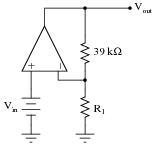• #### Question 13

Calculate the necessary resistor value (R1) in this circuit to give it a voltage gain of 10.5: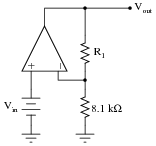• #### Question 14

Predict how the operation of this operational amplifier circuit will be affected as a result of the following faults. Consider each fault independently (i.e. one at a time, no multiple faults):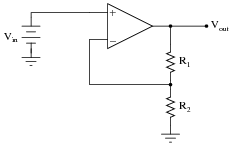Resistor R1 fails open:
Solder bridge (short) across resistor R1:
Resistor R2 fails open:
Solder bridge (short) across resistor R2:
Broken wire between R1/R2 junction and inverting opamp input:

For each of these conditions, explain why the resulting effects will occur.

• #### Question 15

Calculate the voltage gain for each stage of this amplifier circuit (both as a ratio and in units of decibels), then calculate the overall voltage gain:• #### Question 16

Calculate the voltage gain for each stage of this amplifier circuit (both as a ratio and in units of decibels), then calculate the overall voltage gain:• #### Question 17

How much effect will a change in the op-amp’s open-loop voltage gain have on the overall voltage gain of a negative-feedback circuit such as this?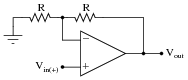If the open-loop gain of this operational amplifier were to change from 100,000 to 200,000, for example, how big of an effect would it have on the voltage gain as measured from the non-inverting input to the output?

• #### Question 18

Suppose a technician is checking the operation of the following electronic circuit: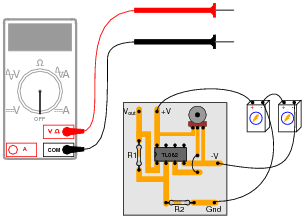She decides to measure the voltage on either side of resistor R1 with reference to ground, and obtains these readings: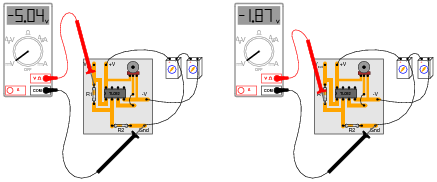On the top side of R1, the voltage with reference to ground is -5.04 volts. On the bottom side of R1, the voltage with reference to ground is -1.87 volts. The color code of resistor R1 is Yellow, Violet, Orange, Gold. From this information, determine the following:

Voltage across R1 (between top to bottom):
Polarity ( and -) of voltage across R1:
Current (magnitude) through R1:
Direction of current through R1:

Additionally, explain how this technician would make each one of these determinations. What rules or laws of electric circuits would she apply?

• #### Question 19

Trace the directions for all currents in this circuit, and calculate the values for voltage at the output (Vout) and at test point 1 (VTP1) for several values of input voltage (Vin):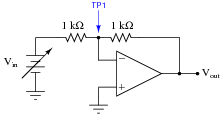Vin VTP1 Vout 0.0 V 0.4 V 1.2 V 3.4 V 7.1 V 10.8 V

Then, from the table of calculated values, determine the voltage gain (AV) for this amplifier circuit.

• #### Question 20

Calculate the overall voltage gain of this amplifier circuit (AV), both as a ratio and as a figure in units of decibels (dB). Also, write a general equation for calculating the voltage gain of such an amplifier, given the resistor values of R1 and R2:• #### Question 21

Calculate all voltage drops and currents in this circuit, complete with arrows for current direction and polarity markings for voltage polarity. Then, calculate the overall voltage gain of this amplifier circuit (AV), both as a ratio and as a figure in units of decibels (dB):• #### Question 22

Determine both the input and output voltage in this circuit:• #### Question 23

The equation for voltage gain (AV) in a typical inverting, single-ended opamp circuit is as follows:

 AV = R1 R2

Where,

R1 is the feedback resistor (connecting the output to the inverting input)

R2 is the other resistor (connecting the inverting input to voltage signal input terminal)

Suppose we wished to change the voltage gain in the following circuit from 3.5 to 4.9, but only had the freedom to alter the resistance of R2:Algebraically manipulate the gain equation to solve for R2, then determine the necessary value of R2 in this circuit to give it a voltage gain of 4.9.

• #### Question 24

Calculate the necessary resistor value (R1) in this circuit to give it a voltage gain of 15: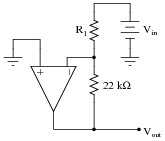• #### Question 25

Calculate the necessary resistor value (R1) in this circuit to give it a voltage gain of 7.5:• #### Question 26

Predict how the operation of this operational amplifier circuit will be affected as a result of the following faults. Consider each fault independently (i.e. one at a time, no multiple faults):Resistor R1 fails open:
Solder bridge (short) across resistor R1:
Resistor R2 fails open:
Solder bridge (short) across resistor R2:
Broken wire between R1/R2 junction and inverting opamp input:

For each of these conditions, explain why the resulting effects will occur.

• #### Question 27

There is something wrong with this amplifier circuit. Note the relative amplitudes of the input and output signals as measured by an oscilloscope: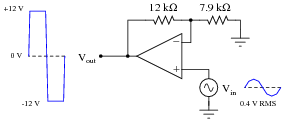This circuit used to function perfectly, but then began to malfunction in this manner: producing a “clipped” output waveform of excessive amplitude. Determine the approximate amplitude that the output voltage waveform should be for the component values given in this circuit, and then identify possible causes of the problem and also elements of the circuit that you know cannot be at fault.

• #### Question 28

Calculate the output voltage of this op-amp circuit (using negative feedback):Also, calculate the DC voltage gain of this circuit.

• #### Question 29

Calculate the voltage gain for each stage of this amplifier circuit (both as a ratio and in units of decibels), then calculate the overall voltage gain:• #### Question 30

Calculate the voltage gain for each stage of this amplifier circuit (both as a ratio and in units of decibels), then calculate the overall voltage gain: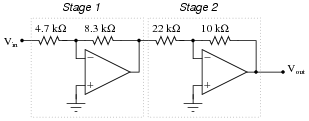• #### Question 31

What possible benefit is there to adding a voltage buffer to the front end of an inverting amplifier, as shown in the following schematic?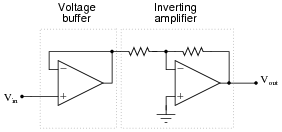• #### Question 32

Operational amplifier circuits employing negative feedback are sometimes referred to as “electronic levers,” because their voltage gains may be understood through the mechanical analogy of a lever. Explain this analogy in your own words, identifying how the lengths and fulcrum location of a lever relate to the component values of an op-amp circuit:• #### Question 33

Compare and contrast inverting versus non-inverting amplifier circuits constructed using operational amplifiers: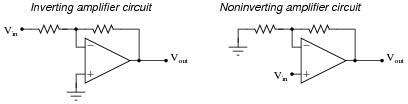How do these two general forms of opamp circuit compare, especially in regard to input impedance and the range of voltage gain adjustment?

• #### Question 34

Predict how the input impedance (Zin) of this inverting operational amplifier circuit will be affected as a result of the following faults. Consider each fault independently (i.e. one at a time, no multiple faults):Resistor R1 fails open:
Solder bridge (short) across resistor R1:
Resistor R2 fails open:
Solder bridge (short) across resistor R2:
Broken wire between R1/R2 junction and inverting opamp input:
Operational amplifier loses power:

For each of these conditions, explain why the input impedance changes as it does.

• #### Question 35

Calculate the voltage gain for each stage of this amplifier circuit (both as a ratio and in units of decibels), then calculate the overall voltage gain: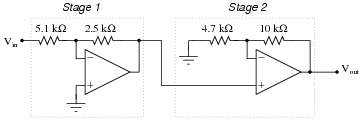• #### Question 36

Shown here are two different voltage amplifier circuits with the same voltage gain. Which of them has greater input impedance, and why? Try to give as specific an answer for each circuit’s input impedance as possible.• #### Question 37

A simple “follower” circuit that boosts the current-output ability of this non-inverting amplifier circuit is a set of bipolar junction transistors, connected together in a “push-pull” fashion like this:However, if connected exactly as shown, there will be a significant voltage error introduced to the opamp’s output. No longer will the final output voltage (measured across the load) be an exact 3:1 multiple of the input voltage, due to the 0.7 volts dropped by the transistor in active mode: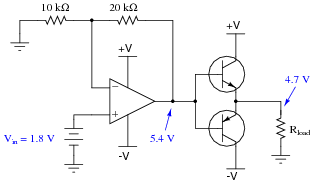There is a very simple way to completely eliminate this error, without adding any additional components. Modify the circuit accordingly.

• #### Question 38

A student wishes to build a variable-gain amplifier circuit using an operational amplifier and a potentiometer. The purpose of this circuit is to act as an audio amplifier for a small speaker, so he can listen to the output of a digital audio player without having to use headphones: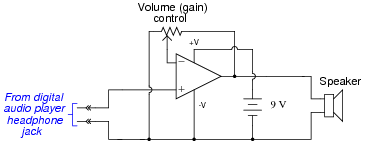Before building the project in a finalized form, the student prototypes it on a solderless breadboard to make sure it functions as intended. And it is a good thing he decided to do this before wasting time on a final version, because it sounds terrible!

When playing a song, the student can hear sound through the headphones, but it is terribly distorted. Taking the circuit to his instructor for help, the instructor suggests the following additions: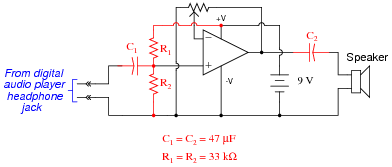After adding these components, the circuit works great. Now, music may be heard through the speaker with no noticeable distortion.

Explain what functions the extra components perform, and why the circuit did not work as originally built.

• #### Question 39

There is something wrong with this amplifier circuit. Despite an audio signal of normal amplitude detected at test point 1 (TP1), there is no output measured at the Äudio signal out” jack: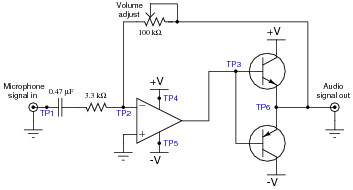Next, you decide to check for the presence of a good signal at test point 3 (TP3). There, you find 0 volts AC and DC no matter where the volume control is set.

From this information, formulate a plan for troubleshooting this circuit, answering the following questions:

What type of signal would you expect to measure at TP3?
What would be your next step in troubleshooting this circuit?
Are there any elements of this circuit you know to be working properly?
What do you suppose would be the most likely failure, assuming this circuit once worked just fine and suddenly stopped working all on it’s own?
• #### Question 40

The junction between the two resistors and the inverting input of the operational amplifier is often referred to as a virtual ground, the voltage between it and ground being (almost) zero over a wide range of circuit conditions: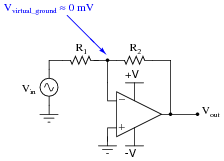If the operational amplifier is driven into saturation, though, the “virtual ground” will no longer be at ground potential. Explain why this is, and what condition(s) may cause this to happen.

Hint: analyze all currents and voltage drops in the following circuit, assuming an opamp with the ability to swing its output voltage rail-to-rail.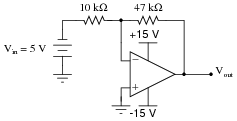• #### Question 41

The same problem of input bias current affecting the precision of opamp voltage buffer circuits also affects non-inverting opamp voltage amplifier circuits: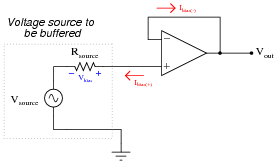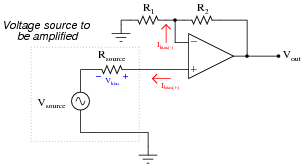To fix this problem in the voltage buffer circuit, we added a “compensating” resistor: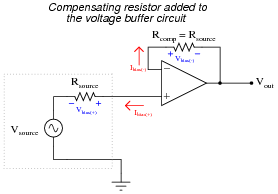To fix the same problem in the non non-inverting voltage amplifier circuit, we must carefully choose resistors R1 and R2 so that their parallel equivalent equals the source resistance:

 R1 || R2 = Rsource

Of course, we must also be sure the values of R1 and R2 are such that the voltage gain of the circuit is what we want it to be.

Determine values for R1 and R2 to give a voltage gain of 7 while compensating for a source resistance of 1.45 kΩ.